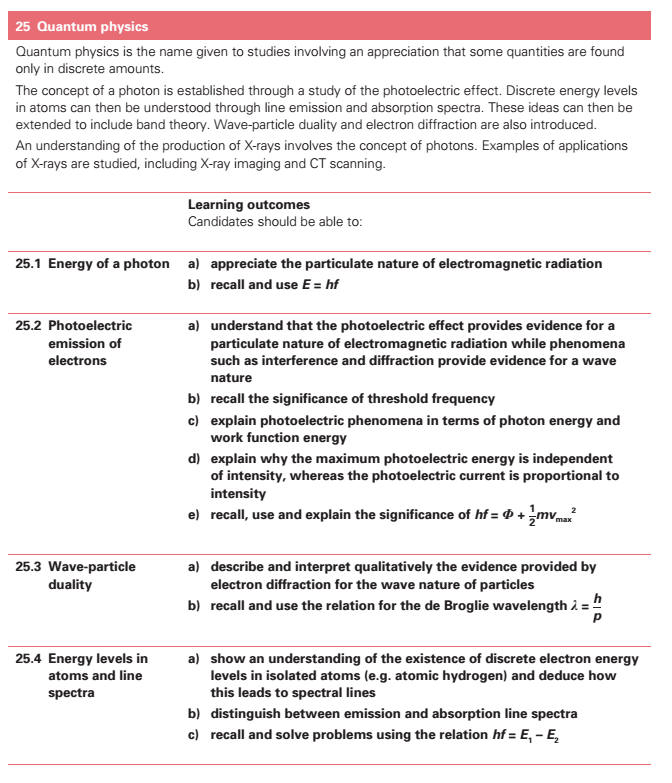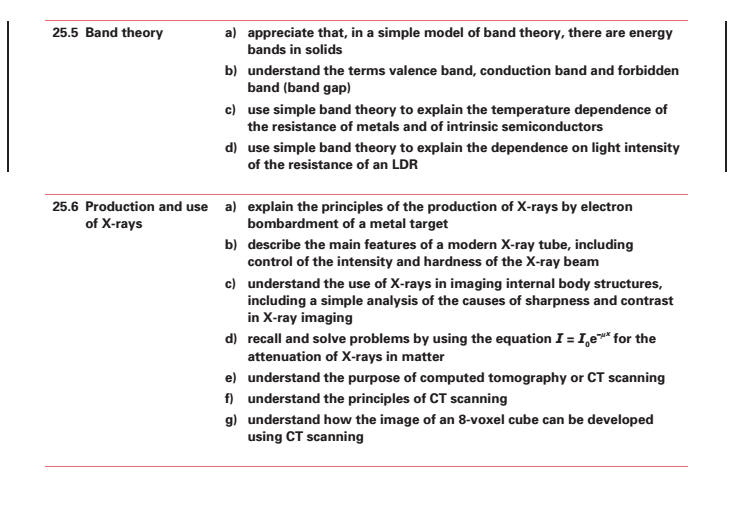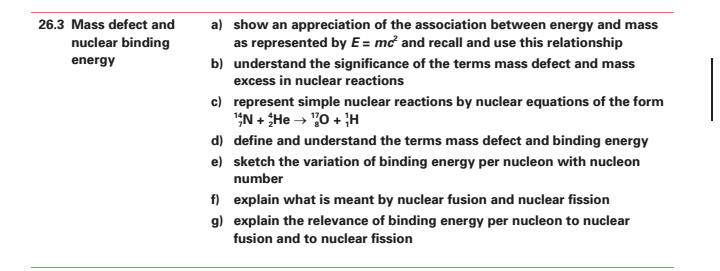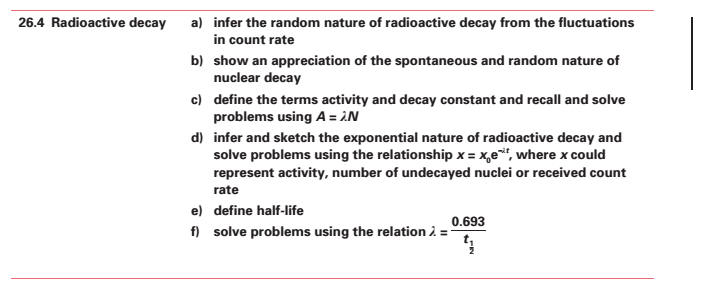26 Particle and Nuclear Physics (k) show an appreciation of the association between energy and mass as represented by E = mc2 and recall and solve problems using this relationship. (l) sketch the variation of binding energy per nucleon with nucleon number. (m) explain the relevance of binding energy per nucleon to nuclear fusion[] and to nuclear fission[]. (n) define the terms activity[] and decay constant[] and recall and solve problems using A = λN. * (o) infer and sketch the exponential nature of radioactive decay and solve problems using the relationship x = xoexp(-λt) where x could represent activity, number of undecayed particles or received count rate. (p) define half-life. (q) solve problems using the relation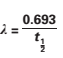.